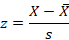# Z-Scores: Home

Z-Scores

What is a Z-Score?

A Z-score is the distance between a certain score and the mean of all scores, and this is measured in standard deviation units.

Why look at Z-Scores?

Z scores allow for comparisons of different scores and distributions to a normal distribution since any data set can be converted to Z-Scores. It also allows for you to determine the probability that a score fits within one standard deviation. Z scores do this by standardizing scores into a standard normal distribution—one with a mean of zero and the standard deviation is 1. So for instance, it can tell you how a score compares to an average population’s mean.

How to Calculate a Z-Score:

Z scores can be calculated by finding the difference between the mean and the actual score, and dividing it by the standard deviation. This is expressed using the following formula:A score of zero means that the score is exactly average, while a score of 1.8 is higher than average. A score of -1.8 means that it would be below average.

How Z-Scores are Used

You can use a Z-table to determine the percentage of scores being higher or lower than the average. For instance, if you have a score that is higher than average, you can use the table to find out the percentage of scores that are higher or lower than the particular score.